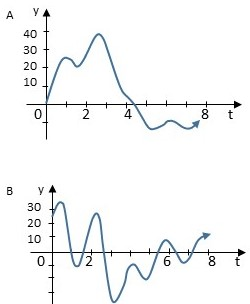# Tell which graph represents the velocity and which represents the distance from a starting point....

## Question:

Tell which graph represents the velocity and which represents the distance from a starting point. (Hint: consider where the derivative is zero, positive, or negative.)## Function and Derivative Graph:

Between the graph of a function and its derivative there is the following relation:

1. the graph of the derivative is positive when the function is increasing.

2. negative, when the function is decreasing

3. and zero in the values of the independent variable where the local ends of the function are reached

## Answer and Explanation:

Considering where the derivative is zero, positive, or negative is not difficult too identify the graph A with the function and the graph B with the derivative.

#### Learn more about this topic:Graphs: Types, Examples & Functions

from High School Algebra II: Help and Review

Chapter 16 / Lesson 11
355K